## Reporting Correlation Coefficient Results and Plots: A SAS® Macro That Does It All

Started ‎04-13-2021 by
Modified ‎05-13-2021 by
Views 2,940
Paper 1163-2021
Authors

Zhengming Chen, Weill Cornell Medicine;

Bowen (Charles) Zheng, Episcopal High School

## Abstract

In academic and particularly medical research, the essential first set of results are often summarized in a table called Table-1. Table-1 is an informative cross table that consists of a set of numerical and categorical variables in rows stratified by a categorical variable in the columns. This single table provides the appropriate summary statistics for all numerical and categorical variables assessed in the study, as well as their bivariate associations with the categorical variable that they are stratified by. There are many user-written SAS macros dedicated to producing such a Table-1 with minimal effort. However, these cross tables cannot provide the bivariate associations between two numerical variables. To supplement these Table-1s, there is a SAS macro that produces a publication-ready document that reports in a single table. This document contains the summary statistics of numerical variables, an appropriate correlation coefficient between two numerical variables with its confidence interval, and the p-value from hypothesis testing for association. To assist in the interpretation of results, it can also optionally produce footnotes and publication-quality figures to visualize the relationship between variables. This SAS macro is simple and easy to use, and it provides a one-stop, powerful tool for all types of correlation studies between two numerical variables. It has been fully tested in different SAS interfaces and is incorporated with the SAS ODS system.

# INTRODUCTION

In medical research, we often provide a single table called Table-1 to summarize the characteristics of our study subjects, such as patients’ age, sex and lab test results. This table is often a cross-table stratified by study groups, for example, medical treatments; and a range of bivariate associations between each of the patients’ characteristics and the stratifying group can be incorporated into this table as well. Depending on the types of characteristics, the strength of a bivariate association can be mean difference, odds ratio, or percent difference, etc. The statistical hypothesis tests from which the p-values are computed can be Student’s t-test, Wilcoxon Rank-sum test, or Chi-squared test (or Fisher’s exact test), etc. This Table-1 is thus very informative and often essential for a medical research paper1,2. Moreover, researchers are often interested in another type of bivariate relationship -- between two numerical measurements, such as how a patient’s age is related to the titer of Covid-19 antibody or how a patient’s BMI is related to the severity of Covid-19 symptoms, etc. These types of questions are commonly addressed by correlations and the related hypothesis testing.

Correlation is a monotonic (often linear) association between two random variables X and Y. The random variables can take either discrete or continuous numerical values3,4,5. The strength and the direction of the correlation are measured by a signed numerical metric called Correlation Coefficient (r). Depending on the types of values and their underlying distribution, different types of correlation coefficients are developed. The most common type of correlation coefficients is Pearson Correlation Coefficient (i.e. Pearson Product-moment Correlation Coefficient, Pearson’s r). It is a parametric measure of correlation and can be defined as the covariance of two variables divided by the product of their standard deviations. It is denoted by the Greek letter ρ (Rho, the corresponding letter of R for the term Regression), and if applied to population, the computation formula is:

where µx and µy are population means of X and Y, respectively; σx and σy are the population standard deviations of X and Y, respectively.

The population statistics are often unknown so they are estimated from the samples. The sample correlation coefficient is denoted by r and can be calculated as:

where  and  are the sample means of  and , respectively;  and  are sample standard deviations of  and , respectively. Note that the  is used instead of  when estimating the correlation coefficient from the samples.

In order to properly infer the strength of a correlation coefficient in the population from samples, two random variables need to follow bivariate (jointly) normal distribution. When this assumption is violated, a non-parametric Spearman Correlation Coefficient (Spearman Rank-order Correlation Coefficient) can be considered. It is calculated as the Pearson Correlation Coefficient but uses the data ranks instead of their actual values. Therefore, a monotonic but non-linear bivariate relationship can be assessed. It is also robust against outliers because of this.

Another related type of correlation, Polychoric Correlation, is also commonly used when two observed variables are ordinal with finite sets of discrete values but are thought to be derived from unobserved variables with a bivariate normal distribution. The Polychoric Correlation Coefficient is the maximum likelihood estimate of the Pearson Correlation Coefficient. When one variable is ordinal but it represents an unobserved continuous variable, and the other variable is a directly observed continuous variable, then a Polyserial Correlation can be used. It is computed through maximum likelihood estimation of a set of parameters. Polychoric and Polyserial correlations are frequently applied to data obtained from survey instruments with Likert scales, as well as classification of diseases with ordinal values.

All types of correlation coefficients lie between -1 and 1, with 1 indicating a perfectly positive correlation and -1 indicating a perfectly negative correlation. The closer the correlation coefficient is to -1 or 1, the stronger the correlation is, with 0 indicating no linear relationship. There are rule-of-thumbs to classify the correlation coefficients into descriptors to aid the interpretation of the strength of correlation. One of those classifies the correlation as very weak if the absolute correlation coefficient is 0.00 – 0.19; weak if it is 0.20 – 0.39; moderate if 0.40 – 0.59; strong if 0.60 – 0.79, and very strong if 0.80 – 1.00.

We are often not only interested in the strength of correlation, but also the probability that it differs from a pre-specified one in order to make an inference, or more preferably, the confidence range surrounding the correlation coefficient. This is achieved through hypothesis testing under a specified null hypothesis after a Fisher’s z-transformation.

As mentioned above, correlation coefficient is closely related to linear regression. In fact, Pearson Correlation Coefficient r can be delivered from a simple linear regression: the square root of its R2 with a sign in front of it. However, one should note that the assumptions and interpretation of r and R2 are different.

There are many other ways to think about a correlation coefficient6. Rick Wicklin from SAS wrote a nice blog7 in 2017 describing seven of those and he provided SAS codes to implement them, including a scatter plot of two variables; the slope of a regression line on the standardized values; the angle between two vectors, etc.

As described at the beginning, a Table-1 provides a wealth of information about a study, and there are many user-written SAS Macros to produce such Table-12. However, Table-1 is a cross-table stratified by a categorical variable, so a correlation coefficient between two numerical variables and its hypothesis testing result cannot be incorporated into this table. Therefore, a different table which provides summary statistics and bivariate association between two numerical variables is needed to supplement the Table-1. This paper describes the development of a SAS Macro - %CorrReport that computes different types of correlation coefficient and the related hypothesis testing results, and reports them in a single table with optional figures.

# SAS IMPLEMENTATION OF CORRELATION ANALYSIS

A single SAS procedure -- PROC CORR offers parametric and non-parametric correlation coefficient analyses. The types of correlation include:

• Pearson product-moment correlation (parametric)
• Spearman rank-order correlation (non-parametric)
• Kendall’s tau-b coefficient (non-parametric)
• Hoeffding’s measure of dependence, D (non-parametric)
• Pearson, Spearman, and Kendall partial correlation
• Polychoric correlation
• Polyserial correlation

The basic syntax is as follows:

``````PROC CORR data = sashelp.class;
var height;
with age weight;
RUN;``````

This will by default, compute the Pearson product-moment correlation between the variable height and each of the variables age and weight. By default, the results appeared in Output Delivery System (ODS) include a table with 6 summary statistics; a table with correlation coefficient and the p-value from hypothesis testing under the null hypothesis ρ = 0, as shown in Fig. 1: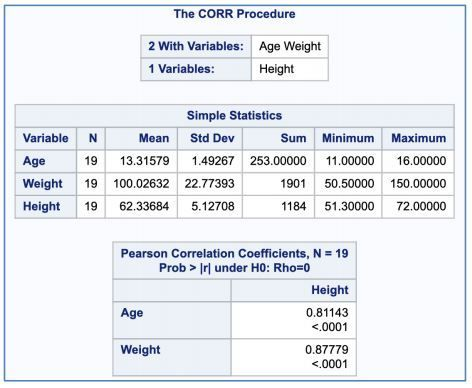### Figure 1. Default table output

If to omit the with statement, it will compute statistics for every pair of variables listed in the var statement.

Two types of plots can be requested like following:

``````PROC CORR data = sashelp.class plots = all;
var height;
with age weight;
RUN;``````

This will produce individual scatter plots with 95% prediction ellipse and a single scatter plot matrix (Fig. 2):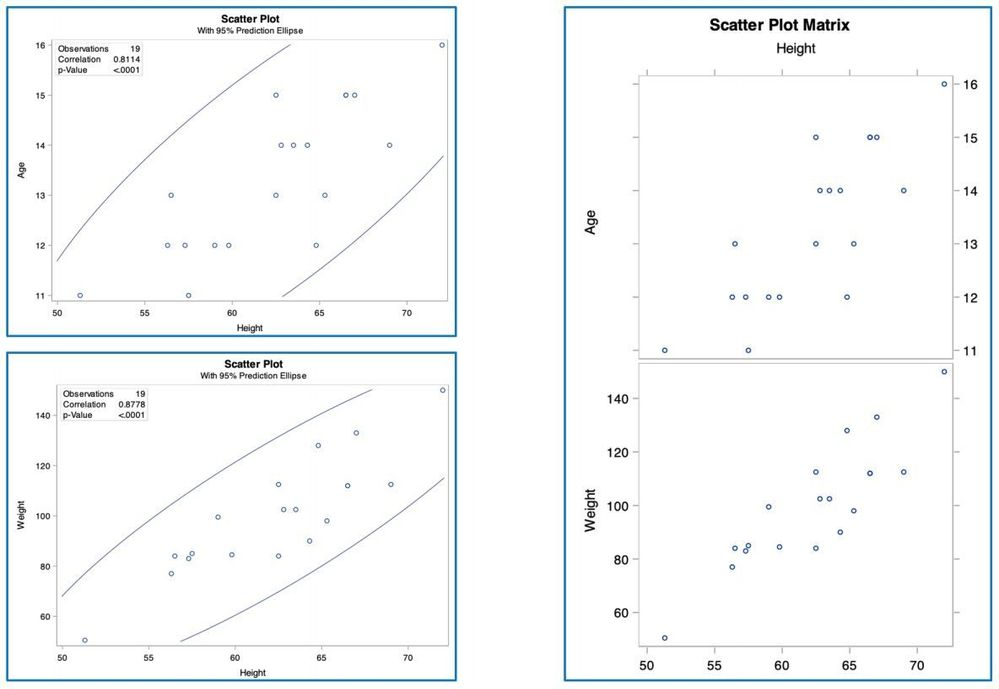### Figure 2. Default plot output

To request different types of correlation coefficient, use a Proc option from the list of spearman | hoeffding | kendall | polychoric | polyserial like this:

``````PROC CORR data = sashelp.class spearman;
var height;
with age weight;
RUN;``````

It is often preferable to report the confidence interval of a correlation coefficient. This can only be computed after a Fisher’s z-transformation. You can request this with fisher Proc option. You can also change the default null hypothesis with rho= within this option. Here are requesting the 95% confidence interval of Pearson’s r and the p-value testing the null hypothesis of ρ = 0.2:

``````PROC CORR data = sashelp.class fisher(rho=0.2);
var height;
with age weight;
RUN;``````

After running these, you will see the requested results in an additional table (Fig. 3):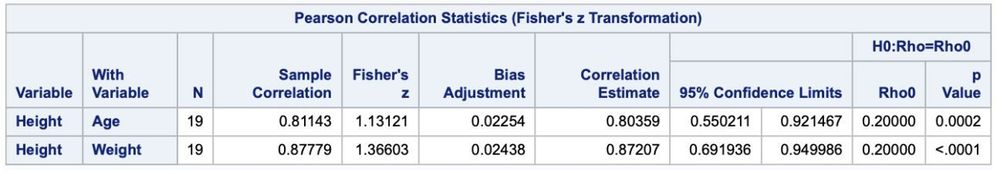# A SAS MACRO TO REPORT CORRELATION ANALYSIS RESULTS

As illustrated above, different parts of results produced by the Proc CORR, as with any other pieces of SAS code, will be in separate tables appeared in ODS output. You can choose to use ODS SAS codes to report the raw tables directly. However, one often wants to report them in a more organized way – a single table in a publication-ready format, preferably with minimum and standard input. This requires series of Data Step to reorganize the results with notations and then use a reporting tool such as Proc REPORT to report them in a desired format. If needed, a plot can also be included, obtained either directly from Proc CORR or after being customized from using a Graphics Proc such as Proc SGPLOT. All these steps can be further packed into a SAS Macro so the SAS codes can be used in an efficient and dynamic way.

## STRATEGY AND WORKFLOW TO BUILD THE SAS MACRO

The chart below (Fig. 4) illustrates the strategy and workflow to build the SAS Macro %CorrReport: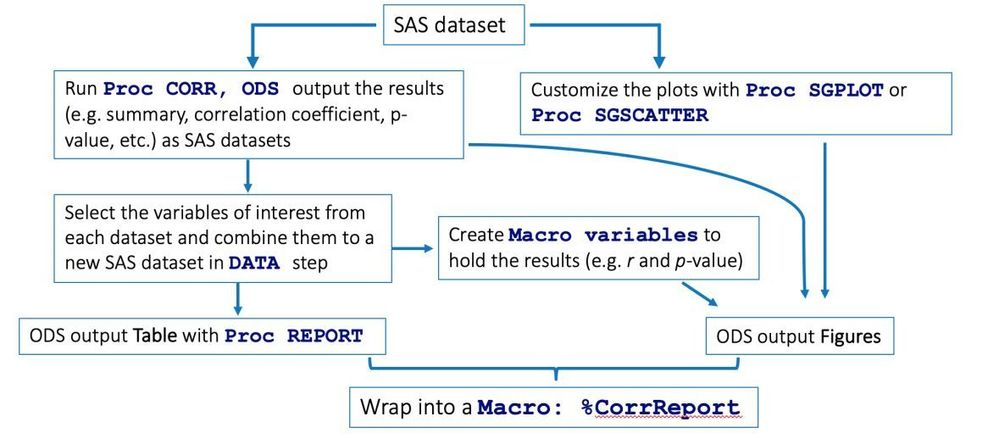## USE THE MACRO

The resulting SAS Macro has three required parameters (Fig. 5): Dataset - dataset name; V1 - name of one of the two numerical variables; V2 - name(s) of the other variable(s). Optional parameters include: 1) type - name of the type of correlation. You can choose from one of these: Pearson, Spearman, Polychoric or Polyserial. The default is to produce results from both Pearson and Spearman correlation analyses. 2) H0 - Null hypothesis. Specify the ρ value for null hypothesis. The default is 0. 3) plot - To produce plot to visualize the correlation. You can choose from regression, ellipse or no. The default is no plot. A regression plot is a customized scatter plot which includes the regression line, its confidence band and a statistics inset. When there are more than one variable names in parameter V2, the plot is a panel with each scatter plot. 4) V1label, V2label and legendPosition – if a regression  plot is requested and only one variable name in V2, you can further change the label on its X-axis, Y-axis, and the position of the statistics inset in the plot for publication quality.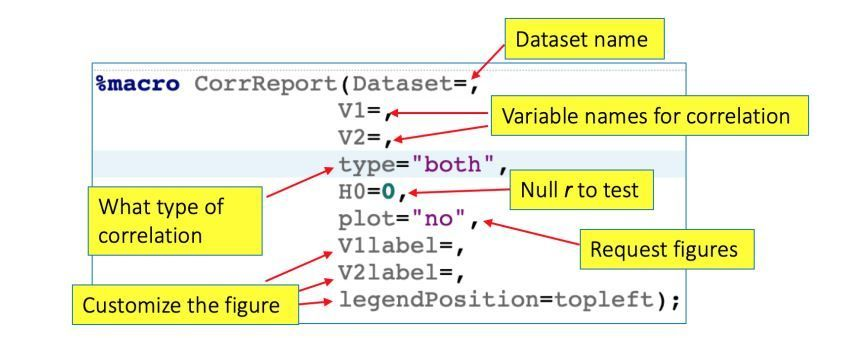### Figure 5. Parameters of the SAS Macro

An example report as a rtf file from running the Demo codes (see Appendix) includes tables such as the one below (Fig. 6). In this single table, you will see the summary statistics of each variable, the correlation coefficient with the 95% confidence interval, the p-value, and the footnotes that annotate the table, including a rule-of-thumb for interpretation of the r. The p-value will be highlighted in red if it is < 0.05.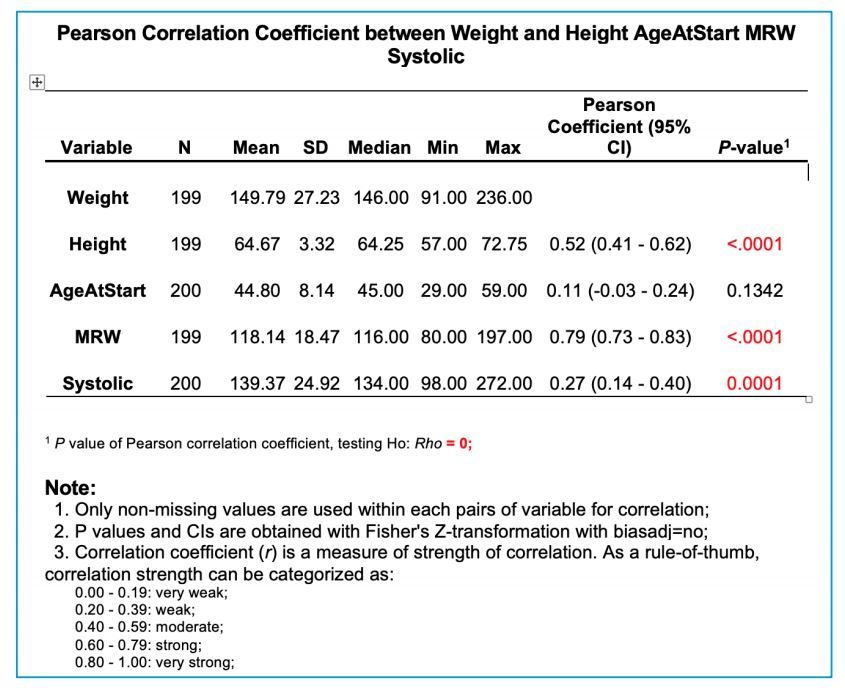### Figure 6. An example table from running the SAS Macro

An example plot as seen in Fig. 7 shows a publication-quality scatter plot superimposed by a regression line and its confidence band. The inset dynamically reports the r and the corresponding p-value.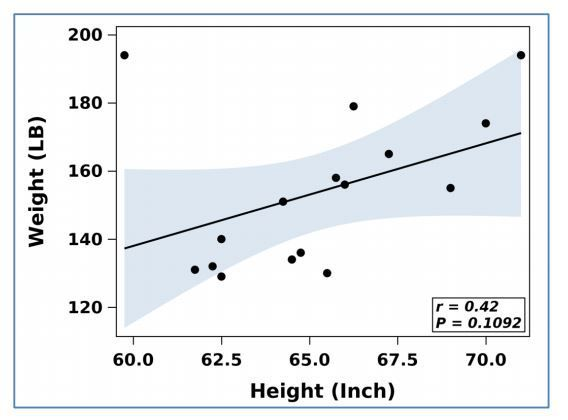# CONCLUSIONS

We have developed a SAS Macro %CorrReport to perform different types of correlation coefficient analyses and report results in a publication-ready format with tables and figures. It is an easy-to-use tool that dramatically enhances the productivity and reproducibility of your research. Moreover, the process of building this Macro serves as an example of developing a SAS Macro from scratch, hence a good case for educational purposes.

# LIMITATIONS

As for now, the limitations of this Macro includes: there are no customized error or warning messages incorporated yet; other uncommon types of correlation, such as Kendall’s tau-b, Hoeffding’s D, and partial correlation, are yet to be included. These can be easily added in a later version.

# REFERENCES

1. Joyner MJ, Carter RE, Senefeld JW, et.al. 2021. “Convalescent Plasma Antibody Levels and the Risk of Death from Covid-19.” Engl J Med., 384(11):1015-1027.
2. Gan, Geliang. 2019. “Create publication-ready variable summary table using SAS macro.” PharmaSUG 2019 Conference Proceedings, BP-175. Cary, NC: SAS Institute Inc.
3. Bewick, Viv. Cheek, Liz. Ball, Jonathan. 2003. “Statistics review 7: Correlation and regression.” Critical Care, 7:451-459.
4. Schober, Patrick. Boer, Christa. Schwarte, Lothar. 2018. “Correlation Coefficients: Appropriate Use and Interpretation.” Anesthesia & Analgesia, 126(5):1763-1768.
5. Rosner, Bernard. 2010. Fundamentals of Biostatistics. Boston, MA: Brooks/Cole.
6. Rodgers Lee, Joseph. Nicewander, W. Alan. 1988. “Thirteen Ways to Look at the Correlation Coefficient.” The American Statistician. 42(1):59-66.
7. Wicklin, Rick. 2017. “7 ways to view correlation.” Accessed April 16, 2021. https://blogs.sas.com/content/iml/2017/09/05/7-ways-view-correlation.html

# ACKNOWLEDGMENTS

We thank The SAS Global Forum 2021 Conference Team for the invitation to present this work.

• Base SAS® Procedures Guide – Statistical Procedures, Sixth Edition. Cary, NC, USA: SAS Institute Inc.
• Carpenter, Art. 2016. Carpenter's Complete Guide to the SAS Macro Language, Third Edition. Cary, NC, USA: SAS Institute Inc.

# CONTACT INFORMATION

Your comments and questions are valued and encouraged. Contact the author at:

Zhengming Chen

Weill Cornell Medicine

zhc2006@med.cornell.edu

Bowen (Charles) Zheng

Episcopal High School

bzheng22@episcopalhighschool.org

# APPENDIX

SAS codes:

``````
/*======= Demo how to use %CorrReport SAS Macro:  ========================*/

/*--- Log: ---------------------------------------------------------------*\

Author:
Zhengming Chen
Date:
April 2021

\*------------------------------------------------------------------------*/

/****** Prepare data: *****************************************************/

/*--- Use the build-in data, and create some ordinal variables: ----------*/
data Demo;
set sashelp.heart(obs=200); /* use the first 200 obs for demo */

/* Cholesterol Level: */
if Chol_Status = "Borderline" then Chol_Level = 2;
else if Chol_Status = "Desirable" then Chol_Level = 1;
else if Chol_Status = "Hight" then Chol_Level = 3;
label Chol_Level = "Cholesterol Level";

/* Smoking Level: */
if Smoking_Status = "Heavy (16-25)" then Smoking_Level = 3;
else if Smoking_Status = "Light (1-5)" then Smoking_Level = 1;
else if Smoking_Status = "Moderate (6-15)" then Smoking_Level = 2;
else if Smoking_Status = "Non-smoker" then Smoking_Level = 0;
else if Smoking_Status = "Very Heavy (> 25)" then Smoking_Level = 4;
label Smoking_Level = "Smoking Level";
run;

/****** Report correlation coefficients and plots: ************************/

/*------------ Call the Macro: -------------------------------------------*/
%include "/folders/SASGF21/Correlation analysis and reporting SAS Macro -CorrReport.sas";

/*------------ Report in a .rtf format: ----------------------------------*/
/* Can set the margin for report this way:       */
OPTIONS center orientation = portrait
topmargin = '1in' bottommargin = '1in'
leftmargin = '1in' rightmargin = '1in';

ods rtf file="
/folders/myshortcuts/Dropbox/zhc2006/SASGF21/
Statistical Analysis Report - Correlation coefficient.rtf"
image_dpi=300 STARTPAGE=no;* style=journal;

ods escapechar = '~';

ods rtf text = "~S={outputwidth=100% just=center}";
ods rtf text = "~S={outputwidth=100% just=center
font=('arial', 18pt,bold)}
Project: Correlation coefficient analysis";
ods rtf text = " ";
ods rtf text = "~S={just=center font =('arial', 12pt)}
- Last updated on: &sysdate9";

ods rtf text = "~S={outputwidth=100% just=center
font=('arial', 11pt)}  ";
ods rtf text = "~S={outputwidth=100% just=left
font=('arial', 16pt,bold)}
Results:  ";
ods rtf text = "~S={outputwidth=100% just=center
font=('arial', 11pt)}  ";

/*--- 1. Pearson correlation coefficient between &V1 and only one variable
in &V2: -------*/
ods rtf startpage=now;
ods rtf text = "~S={leftmargin = 0.1in outputwidth=100% just=center
font=('arial', 11pt)}  ";
ods rtf text = "~S={outputwidth=100% just=left
font = ('arial',14pt, bold) color=blue}
Table-1a: Pearson correlation with plot (one variable vs one variable)";
ods rtf text = "~S={leftmargin = 0.1in outputwidth=100% just=center
font=('arial', 11pt)}  ";

ods select Report(persist);

/* with smaller sample (note how the r and 95% CI band of regression line change): */
/* Also want to change the axis labels: */
%CorrReport(dataset=Demo(obs=16), V1=weight, V2=height,
type="pearson",
plot="regression",
V1Label="Weight (LB)", V2Label="Height (Inch)",
legendPosition=bottomright);

/*--- 2. Pearson correlation coefficient between &V1 and each of variable
in &V2 list: ----------*/
ods rtf startpage=now;
ods rtf text = "~S={leftmargin = 0.1in outputwidth=100% just=center
font=('arial', 11pt)}  ";
ods rtf text = "~S={outputwidth=100% just=left
font = ('arial',14pt, bold) color=blue}
Table-1b: Pearson correlation with plot (one variable vs a list of variables)";
ods rtf text = "~S={leftmargin = 0.1in outputwidth=100% just=center
font=('arial', 11pt)}  ";

ods select Report(persist);

%CorrReport(dataset=Demo, V1=Weight,V2=Height AgeAtStart MRW Systolic,
type="pearson",
plot="regression");

/*--- 3. Pearson correlation coefficient between &V1 and only one variable
in &V2 (testing H0: 0.5): --------------*/
ods rtf startpage=now;
ods rtf text = "~S={leftmargin = 0.1in outputwidth=100% just=center
font=('arial', 11pt)}  ";
ods rtf text = "~S={outputwidth=100% just=left
font = ('arial',14pt, bold) color=blue}
Table-1c: Spearman correlation testing Rho = 0.5";
ods rtf text = "~S={leftmargin = 0.1in outputwidth=100% just=center
font=('arial', 11pt)}  ";

/* Change the axis label: */
%CorrReport(dataset=Demo, V1=weight, V2=height,
type="spearman",
H0=0.5,
plot="regression",
V1Label="Weight (LB)", V2Label="Height (Inch)",
legendPosition=topleft);

/*--- 4. Polychoric correlation between &V1 and only one variable
in &V2: --------------*/
ods rtf startpage=now;
ods rtf text = "~S={leftmargin = 0.1in outputwidth=100% just=center
font=('arial', 11pt)}  ";
ods rtf text = "~S={outputwidth=100% just=left
font = ('arial',14pt, bold) color=blue}
Table-2: Polychoric correlation: An ordinal variable vs. ordinal variables
(levels must be <= 20) ";
ods rtf text = "~S={leftmargin = 0.1in outputwidth=100% just=center
font=('arial', 11pt)}  ";
%CorrReport(dataset=Demo, V1=Chol_Level,V2=Smoking_Level,
type="POLYCHORIC");

/*--- 5. Polychoric correlation between &V1 and only one variable
in &V2: --------------*/
ods rtf startpage=now;
ods rtf text = "~S={leftmargin = 0.1in outputwidth=100% just=center
font=('arial', 11pt)}  ";
ods rtf text = "~S={outputwidth=100% just=left
font = ('arial',14pt, bold) color=blue}
Table-3: Polyserial correlation: A continuous variable vs. ordinal variables
(levels must be <= 20)";
ods rtf text = "~S={leftmargin = 0.1in outputwidth=100% just=center
font=('arial', 11pt)}  ";
/* Note V2 here should be the ordinal variable */
%CorrReport(dataset=Demo, V1=AgeAtStart,V2=Smoking_level,
type="POLYSERIAL",
plot="regression");

/*========================================================================*/
ods rtf close;
ods select all;
footnote;
/********************** End of report *************************************/
``````Hello! Is there a way to download this macro? Thank you for any help!!

Kim

Version history
Last update:
‎05-13-2021 01:39 PM
Updated by:Contributors
Article Labels
Article Tags